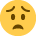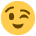# MoP's Height Map / Vertex Blending Shader...Who has it?

Offline / Send Message
polycounter lvl 9
Hey Guys, I've been poking around in max, starting on a piece that would benefit from MoP's shader seen here:

http://www.polycount.com/forum/showpost.php?p=1024948&postcount=54

Does anyone have said shader...the link's brokenMoP! Where are Yoooouuuuu??!

## Replies

• Offline / Send Message
Here you go.
```// Paul "MoP" Greveson's Texture Blend shader!
//
// contact: [email protected]
// website: www.greveson.co.uk
//

// Use correct compiler.
string ParamID = "0x003";

// Light info.
float3 light1Dir : Direction
<
string UIName = "Light 1 Direction";
string Object = "TargetLight";
string UIWidget = "None";
string Space = "World";
int refID = 1;
> = { 100.0f, 100.0f, 100.0f };

float3 light1Pos : POSITION
<
string UIName = "Light 1 Position";
string Object = "PointLight";
string Space = "World";
int refID = 1;
> = { 100.0f, 100.0f, 100.0f };

float4 light1Color : LIGHTCOLOR <int LightRef = 1; string UIWidget = "None"; > = { 1.0f, 1.0f, 1.0f, 1.0f};
float4 light1Attenuation : Attenuation <int LightRef = 1; string UIWidget = "None"; > = { 20.0f, 30.0f, 0.0f, 100.0f};
float light1Hotspot : HotSpot <int LightRef = 1; string UIWidget = "None"; > = { 43.0f };
float light1Falloff : FallOff <int LightRef = 1; string UIWidget = "None"; > = { 45.0f };

#define light1Type 1
#define light1attenType 0
#define light1coneType 0

// Textures.
texture bottomDiffuse
<
string UIName = "Bottom Diffuse";
string ResourceType = "2D";
>;

texture bottomNormal
<
string UIName = "Bottom Normal";
string ResourceType = "2D";
>;

texture bottomSpecular
<
string UIName = "Bottom Specular";
string ResourceType = "2D";
>;

texture topDiffuse
<
string UIName = "Top Diffuse";
string ResourceType = "2D";
>;

texture topNormal
<
string UIName = "Top Normal";
string ResourceType = "2D";
>;

texture topSpecular
<
string UIName = "Top Specular";
string ResourceType = "2D";
>;

texture heightmap
<
string UIName = "Bottom Heightmap";
string ResourceType = "2D";
>;

bool useNormalMaps <
string UIName = "Use Normal Maps";
> = false;

bool useSpecularMaps <
string UIName = "Use Specular Maps";
> = false;

bool flipNormalY <
string UIName = "Invert Normal Map Green Channel";
> = false;

bool useGlossMap <
string UIName = "Derive Gloss from Specular Map Alpha Channel";
> = false;

float glossConstant <
string UIName = "Gloss Constant";
float UIMin = 0.0f;
float UIMax = 1.0f;
float UIStep = 0.01f;
> = 0.3f;

bool useVertexAlpha <
string UIName = "Use Vertex Alpha for Blend Falloff";
> = false;

float falloffConstant <
string UIName = "Blend Falloff Constant";
float UIMin = 0.01f;
float UIMax = 1.0f;
float UIStep = 0.01f;
> = 0.5f;

bool overrideVertexColor <
string UIName = "Override output with Vertex Color";
> = false;

bool overrideVertexAlpha <
string UIName = "Override output with Vertex Alpha";
> = false;

// Samplers.
sampler2D bottomDiffuseSampler = sampler_state
{
Texture = <bottomDiffuse>;
MinFilter = LINEAR;
MagFilter = LINEAR;
MipFilter = LINEAR;
};

sampler2D bottomNormalSampler = sampler_state
{
Texture = <bottomNormal>;
MinFilter = LINEAR;
MagFilter = LINEAR;
MipFilter = LINEAR;
};

sampler2D bottomSpecularSampler = sampler_state
{
Texture = <bottomSpecular>;
MinFilter = LINEAR;
MagFilter = LINEAR;
MipFilter = LINEAR;
};

sampler2D topDiffuseSampler = sampler_state
{
Texture = <topDiffuse>;
MinFilter = LINEAR;
MagFilter = LINEAR;
MipFilter = LINEAR;
};

sampler2D topNormalSampler = sampler_state
{
Texture = <topNormal>;
MinFilter = LINEAR;
MagFilter = LINEAR;
MipFilter = LINEAR;
};

sampler2D topSpecularSampler = sampler_state
{
Texture = <topSpecular>;
MinFilter = LINEAR;
MagFilter = LINEAR;
MipFilter = LINEAR;
};

sampler2D heightmapSampler = sampler_state
{
Texture = <heightmap>;
MinFilter = LINEAR;
MagFilter = LINEAR;
MipFilter = LINEAR;
};

// Vertex Color and Alpha.
int texcoord0 : Texcoord
<
int Texcoord = 0;
int MapChannel = 1;
string UIWidget = "None";
>;

int texcoord1 : Texcoord
<
int Texcoord = 1;
int MapChannel = 1;
string UIWidget = "None";
>;

int texcoord2 : Texcoord
<
int Texcoord = 2;
int MapChannel = 1;
string UIWidget = "None";
>;

int texcoord3 : Texcoord
<
int Texcoord = 3;
int MapChannel = 0;
string UIWidget = "None";
>;

int texcoord4 : Texcoord
<
int Texcoord = 4;
int MapChannel = -2;
string UIWidget = "None";
>;

// Transformations.
float4x4 wvp : WorldViewProjection < string UIType = "None"; >;
float4x4 worldI : WorldInverse < string UIType = "None"; >;
float4x4 worldIT : WorldInverseTranspose < string UIType = "None"; >;
float4x4 viewInv : ViewInverse < string UIType = "None"; >;
float4x4 world : World < string UIType = "None"; >;

// Create the light vector.
float3 lightVec_func(float3 worldSpacePos, float3 lightVector, float3x3 objTangentXf, int lightType)
{
float3 lightVec = mul(objTangentXf, (mul((lightVector - worldSpacePos), worldI).xyz));
return lightVec;
}

// Input from application.
struct a2v
{
float4 position		: POSITION;
float4 tangent		: TANGENT;
float4 binormal		: BINORMAL;
float4 normal		: NORMAL;

float2 texCoord		: TEXCOORD0;
float3 vertexColor	: TEXCOORD3;
float vertexAlpha 	: TEXCOORD4;
};

// Output to fragment program.
struct v2f
{
float4 position    		: POSITION;
float3 lightVec    		: TEXCOORD0;
float3 eyeVec	    	: TEXCOORD1;

float2 texCoord			: TEXCOORD2;
float4 color			: COLOR0;

};

v2f v( a2v In, uniform float3 lightPosition, uniform int lightType, uniform float3 lightDirection )
{
v2f Out = ( v2f )0;
float3x3 objTangentXf;
objTangentXf[ 0 ] = In.binormal.xyz;
objTangentXf[ 1 ] = -In.tangent.xyz;
objTangentXf[ 2 ] = In.normal.xyz;

float3 worldSpacePos = mul( In.position, world ).xyz;
Out.lightVec = lightVec_func( worldSpacePos, lightPosition, objTangentXf, lightType );
float4 osIPos = mul( viewInv[ 3 ], worldI );
float3 osIVec = osIPos.xyz - In.position.xyz;
Out.eyeVec = mul( objTangentXf, osIVec );
Out.position = mul( In.position, wvp );

In.texCoord.y += 1.0f;		// This fixes Max's V texcoord which is off by one!
Out.texCoord = In.texCoord;

float4 vertexColor = float4( In.vertexColor.rgb, In.vertexAlpha.r );
Out.color = vertexColor;

return Out;
}

float4 f( v2f In, uniform float3 lightDir, uniform float4 lightColor, uniform float4 lightAttenuation,
uniform float lightHotspot, uniform float lightFalloff, uniform int lightType, uniform int attenType,
{
float3 ret = float3( 0,0,0 );
float3 V = normalize( In.eyeVec );
float3 L = normalize( In.lightVec );

float4 heightmap = tex2D( heightmapSampler, In.texCoord.xy );
float blendFalloff = falloffConstant;
float4 vertexColor = In.color;
if ( useVertexAlpha )
{
blendFalloff = clamp( vertexColor.a, 0.01, 1.0 );
}
float mixMask = saturate( ( ( heightmap.r - vertexColor.r ) / blendFalloff ) - ( vertexColor.r - 1 ) );

// Diffuse color.
float4 topDiffuse = tex2D( topDiffuseSampler, In.texCoord.xy );
float4 bottomDiffuse = tex2D( bottomDiffuseSampler, In.texCoord.xy );
float3 diffuseColor = lerp( topDiffuse.rgb, bottomDiffuse.rgb, mixMask );

// Specular color.
float3 specularColor = float3( 0, 0, 0 );
if ( useSpecularMaps )
{
float4 topSpecular = tex2D( topSpecularSampler, In.texCoord.xy );
float4 bottomSpecular = tex2D( bottomSpecularSampler, In.texCoord.xy );
specularColor = lerp( topSpecular.rgb, bottomSpecular.rgb, mixMask );
}

// Specular level.
float specularLevel = ( specularColor.r + specularColor.g + specularColor.b ) / 3.0;

// Gloss.
float glossiness = glossConstant;
if ( useGlossMap )
{
float4 topSpecular = tex2D( topSpecularSampler, In.texCoord.xy );
float4 bottomSpecular = tex2D( bottomSpecularSampler, In.texCoord.xy );
glossiness = lerp( topSpecular.a, bottomSpecular.a, mixMask );
}

// Normal.
float3 normal = float3( 0.5, 0.5, 1.0 );
if ( useNormalMaps )
{
float4 topNormal = tex2D( topNormalSampler, In.texCoord.xy );
topNormal.xyz = topNormal.xyz * 2 - 1;
if ( flipNormalY )
{
topNormal.y = -topNormal.y;
}
topNormal.rgb = normalize( topNormal.rgb );
float4 bottomNormal = tex2D( bottomNormalSampler, In.texCoord.xy );
bottomNormal.xyz = bottomNormal.xyz * 2 - 1;
if ( flipNormalY )
{
bottomNormal.y = -bottomNormal.y;
}
bottomNormal.rgb = normalize( bottomNormal.rgb );
normal = normalize( lerp( topNormal.rgb, bottomNormal.rgb, mixMask ) );
}

// Final calculations.
float NdotL = dot( normal, L );				// Calculate the diffuse.
float diffuse = saturate( NdotL );			// Clamp to zero.
diffuseColor *= diffuse;					// The resulting diffuse color.
specularColor *= specularLevel;
float3 H = normalize( L + V );				// Compute the half angle.
float NdotH = saturate( dot( normal, H ) );
specularColor *= pow( NdotH, glossiness );	// Raise to glossiness power and compute final specular color.
ret += specularColor + diffuseColor;
ret *= lightColor;							// Multiply by the color of the light.
float4 done = float4( ret, 1 );				// Create the final output.

// Debug overrides.
if ( overrideVertexColor )
{
done = vertexColor.r, vertexColor.g, vertexColor.b, 1.0;
}
if ( overrideVertexAlpha )
{
done = vertexColor.a, vertexColor.a, vertexColor.a, 1.0;
}

return done;
}

technique Complete
{
pass light1
{
VertexShader = compile vs_2_0 v(light1Pos,  light1Type, light1Dir);
ZEnable = true;
CullMode = cw;
ZWriteEnable = true;
AlphaBlendEnable = false;
SrcBlend = SrcAlpha;
DestBlend = One;
AlphaTestEnable = FALSE;
PixelShader = compile ps_2_0 f(light1Dir, light1Color, light1Attenuation, light1Hotspot, light1Falloff, light1Type, light1attenType, light1coneType, light1CastShadows, 1);
}
}
```
• Offline / Send Message
polycounter lvl 9
Thanks Ben...unfortunately I have no idea how to use that as is• Offline / Send Message
Copy/paste into a blank text document (notepad), then save as whateverFilenameYouWant.fx

I figured it would be better to post the code than link to a file that could someday be lost again.
• Offline / Send Message
polycounter lvl 9
Ah, thanks Ben, yeah I can totally see the reasoning behind it...thanks for your help, it's much appreciated!
• Offline / Send Message

It was my fault for assuming you (or anyone else for that matter) would know what to do with the code. The deeper I get into coding myself I forget what's "common knowledge" and what's not.

I guess it goes without saying even though it's probably obvious at this point, but I'll mention it anyway just to be sure. You can also open up any other shaders you might have access to, since they are usually stored in plain text format and can be easily opened up with notepad, in order to take a look at the code and make modifications.

If you end up doing this a lot you'll probably want to use notepad++ rather than regular notepad which should help make the code easier to read and edit (though it's not necessary). Even better is ShaderFX which is node based like UDK's material editor, though it requires Max.

I think there are a few links to shader tutorials and examples in the Programming Scripting Master Thread if you are interested.
• Offline / Send Message
polycounter lvl 9
No dramas Ben, we're in the technical talk forums after allThanks for the links...if I'm feeling brave I might tinker with the code, it's always interesting seeing what's talking to what and experimenting with code haha.